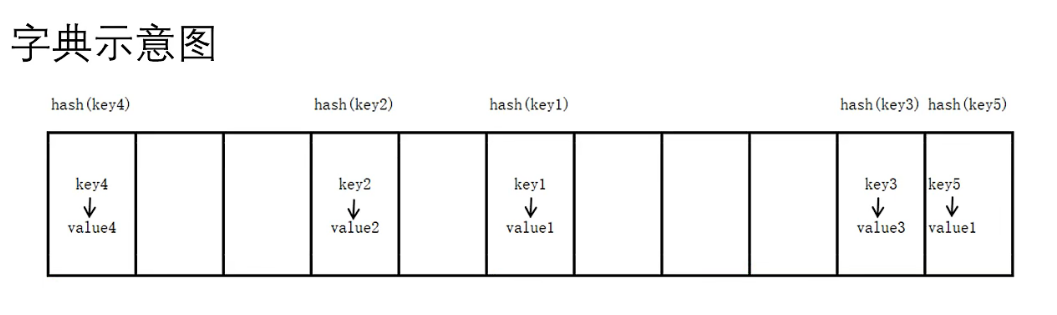### Python中的字典

1. 字典概念

• Python内置的数据结构之一，与列表一样是一个可变序列。

• 以键值对的方式存储数据，字典是一个无序的序列。（列表是有序的）• 字典通过计算key的hash值确定存储位置，所以key应为不可变序列（字符串，整数等）

2. 字典原理

• Python中的字典根据key，通过hash函数，计算出存储位置，找到value

3. 字典的创建

``` """字典的创建方式"""
'''使用{}创建字典'''
scores = {'张三': 100, '李四': 98, '王五': 45}
print(scores)
print(type(scores))
​
'''第二种创建dict()'''
student = dict(name='jack', age=20)
print(student)
​
'''空字典'''
d = {}
print(d)```

4. 字典元素的获取

``` """获取字典的元素"""
scores = {'张三': 100, '李四': 98, '王五': 45}
'''第一种方式，使用[]'''
print(scores['张三'])
# print(scores['陈六'])  # KeyError: '陈六'
​
'''第二种方式，使用get()方法'''
print(scores.get('张三'))
print(scores.get('陈六'))  # None
print(scores.get('赵七', 99))  # 99是在查找'赵七'所对应的value不存在时，提供的一个默认值```

5. 字典的常用操作

``` """字典元素的增删改操作"""
'''key的判断'''
scores = {'张三': 100, '李四': 98, '王五': 45}
print('张三' in scores)
print('张三' not in scores)
​
del scores['张三']  # 删除指定的key-value对
# scores.clear()  # 清空字典的元素
print(scores)
scores['陈六'] = 98  # 新增元素
print(scores)
​
scores['陈六'] = 100  # 修改元素
print(scores)```
``` """获取字典视图"""
scores = {'张三': 100, '李四': 98, '王五': 45}
# 获取所有的key
keys = scores.keys()
print(keys)
print(type(keys))
print(list(keys))  # 将所有的key组成的视图转成列表
​
# 获取所有的value
values = scores.values()
print(values)
print(type(values))
print(list(values))
​
# 获取所有的key-value对
items = scores.items()
print(items)
print(list(items))  # 转化之后的列表元素是由 元组 组成```

6. 字典元素的遍历

``` scores = {'张三': 100, '李四': 98, '王五': 45}
# 字典元素的遍历
for item in scores:
print(item, scores[item], scores.get(item))```

7. 字典的特点

• 字典中的所有元素都是一个 key-value对，key不允许重复，value可以重复

• 字典中的元素是无序的

• 字典中的key必须是不可变对象

``` lst = [10, 20, 30]
d = {lst: 100}
print(d)  # TypeError: unhashable type: 'list'```
• 字典也可以根据需要动态地伸缩

• 字典会浪费较大的内存，是一种使用空间换时间的数据结构

8. 字典生成式

``` items = ['Fruits', 'Books', 'Others']
prices = [96, 78, 85]
d = {item: price for item, price in zip(items, prices)}
print(d)
​
items = ['Fruits', 'Books', 'Others']
prices = [96, 78, 85, 100, 120]
d = {item.upper(): price for item, price in zip(items, prices)}
print(d)```